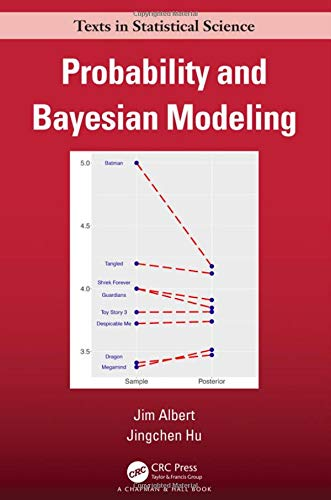# Probability and Bayesian Modeling

• Length: 552 pages
• Edition: 1
• Publisher:
• Publication Date: 2019-12-18
• ISBN-10: 1138492566
• ISBN-13: 9781138492561
Description

Probability and Bayesian Modeling is an introduction to probability and Bayesian thinking for undergraduate students with a calculus background. The first part of the book provides a broad view of probability including foundations, conditional probability, discrete and continuous distributions, and joint distributions. Statistical inference is presented completely from a Bayesian perspective. The text introduces inference and prediction for a single proportion and a single mean from Normal sampling. After fundamentals of Markov Chain Monte Carlo algorithms are introduced, Bayesian inference is described for hierarchical and regression models including logistic regression. The book presents several case studies motivated by some historical Bayesian studies and the authors’ research.

This text reflects modern Bayesian statistical practice. Simulation is introduced in all the probability chapters and extensively used in the Bayesian material to simulate from the posterior and predictive distributions. One chapter describes the basic tenets of Metropolis and Gibbs sampling algorithms; however several chapters introduce the fundamentals of Bayesian inference for conjugate priors to deepen understanding. Strategies for constructing prior distributions are described in situations when one has substantial prior information and for cases where one has weak prior knowledge. One chapter introduces hierarchical Bayesian modeling as a practical way of combining data from different groups. There is an extensive discussion of Bayesian regression models including the construction of informative priors, inference about functions of the parameters of interest, prediction, and model selection.

The text uses JAGS (Just Another Gibbs Sampler) as a general-purpose computational method for simulating from posterior distributions for a variety of Bayesian models. An R package ProbBayes is available containing all of the book datasets and special functions for illustrating concepts from the book.

A complete solutions manual is available for instructors who adopt the book in the Additional Resources section.

Free sampleTry Audible »